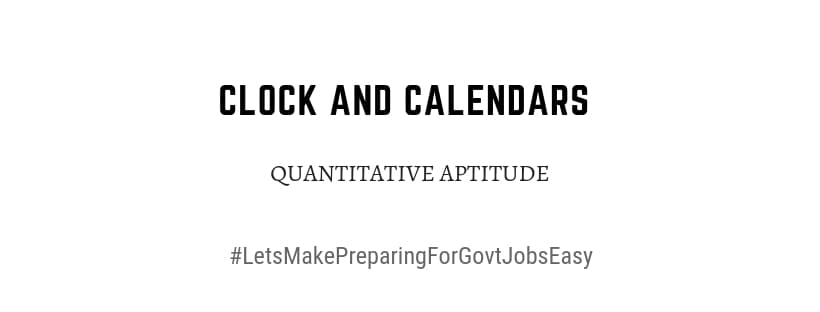Home > Aptitude and Reasoning > CLOCK-CALENDER

# CLOCK-CALENDER# CLOCK CALENDAR QUANTITATIVE APTITUDE WITH PRACTICE PROBLEMS

Aptitude Reasoning eBook

FIND THE DAY OF ANY DATE YEAR

Year is divided into two types

1) Normal (Ordinary) year: The year which is not divisible by 4
Ex: 1983, 1991, 2017

An ordinary year has 365 days

2) Leap Year: The year which is exactly divisible by 4

Ex: 1804, 1920, 2004, 2016
i) A leap year has 366 days
ii) Every 4th century is a leap year and no other century is a leap year
iii) Each of the years 400, 800, 1200, 2000 etc. is a leap year
Odd Days

In a given period, the number of days more than complete weeks are called Odd
Days

COUNTING OF ODD DAYS
1) One ordinary year = 365 days (52 weeks + 1 day)
i.e, One ordinary year has 1 odd day

2) One leap year = 366 days (52 weeks + 2 days)
i.e, One leap year has 2 odd days

3) 100 years = 76 ordinary years + 24 leap years
= (76 × 1 + 24 × 2) odd days
= 124 odd days
= (17 weeks + 5 days) ≡ 5
i.e, Number of odd days in 100 years = 5

See Preview of EBook Recommended Quantitative Aptitude Book in Google Book Play Store